Transformers - 3

# Transformers - 3 Notes | Study Electrical Engineering SSC JE (Technical) - Electrical Engineering (EE)

## Document Description: Transformers - 3 for Electrical Engineering (EE) 2022 is part of Electrical Engineering SSC JE (Technical) preparation. The notes and questions for Transformers - 3 have been prepared according to the Electrical Engineering (EE) exam syllabus. Information about Transformers - 3 covers topics like and Transformers - 3 Example, for Electrical Engineering (EE) 2022 Exam. Find important definitions, questions, notes, meanings, examples, exercises and tests below for Transformers - 3.

Introduction of Transformers - 3 in English is available as part of our Electrical Engineering SSC JE (Technical) for Electrical Engineering (EE) & Transformers - 3 in Hindi for Electrical Engineering SSC JE (Technical) course. Download more important topics related with notes, lectures and mock test series for Electrical Engineering (EE) Exam by signing up for free. Electrical Engineering (EE): Transformers - 3 Notes | Study Electrical Engineering SSC JE (Technical) - Electrical Engineering (EE)
 1 Crore+ students have signed up on EduRev. Have you?

VOLTAGE RECULATION
It is defined as the rise in voltage expressed as a fraction f full load rated voltage, when full load (at specified pf) is removed while keeping input voltage constant.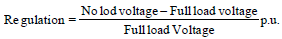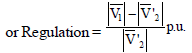where full load voltage = Rated voltage.
V'2 = aV2

Approximate Voltage Regulation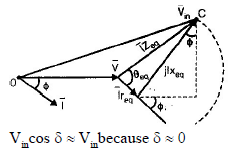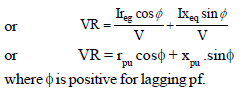To find voltage regulation at leading pf replace ø by –ø.

Condition for Maximum Voltage Regulation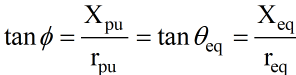• Maximum voltage regulation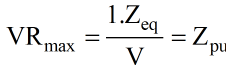Note:
• Here tanø is positive, therefore, maximum voltage regulation occurs at a lagging load

p.f. = req/Zeq

Condition for Zero Voltage Regulation

•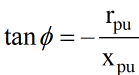or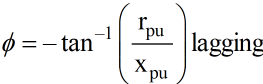or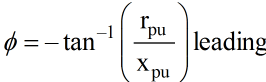or ø = (90°– θeq) leading

• Negative value of tanø indicates a leading pf. Therefore zero voltage regulation occurs when load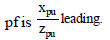Remember:
• For leading pf greater than Xpu/Zpu the voltage regulation will be negative.

TRANSFORMER LOSSES
• There are mainly two kinds of losses in a transformer.
Core Loss
• These consist of hysteresis loss Ph and eddy current loss Pe.
• Hysteresis loss P= Kh fBmx

• Eddy current loss P= Ke f2Bm2

Where,
K= Proportionality constant depend upon the quality of core and volume.
Ke = Proportionality constant whose depends on the volume and resistiviy of the core material, thickness of laminations and the units employed.
Bm = maximum flux density in the core
f = frequency of the alternating flux

x = Steinmetz's constant varies from 1.5 to 2.5. it is also depend upon magnetic material

∴ Pc =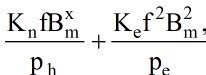• Losses in terms of applied voltage and frequency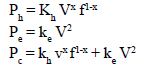Ohmic loss
• Ohmic losses occurs in both the primary and secondary winding resistance.

Pcu = I2req

Note:
• Generally student get confused related with iron loss and copper loss. Actually iron loss (Pi) is also called core loss (Pc) and copper loss (Pcu) is also called ohmic loss (Poh). So it must be clear iron loss is also called constant loss because it is independent to the load current and ohmic loss (I2req ) is called variable loss because it is totally dependent to the square of load current.

EFFICIENCY OF TRANSFORMER
• The efficiency is may be defined as the ratio of output power to input power.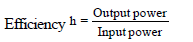or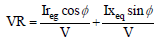Condition for Maximum Efficiency
• Pi = Pcu = I2 req
• Copper loss = Iron loss
• The maximum efficiency for a constant load current occurs at unity power factor.
• Current maximum efficiency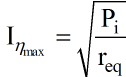• kVA rating at maximum efficiency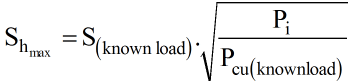Where
S(know load) = kVA at known load
Pcu(know load) = Copper loss at known load

AUTO TRANSFORMER
• A single-phase auto-transformer is a one-winding transformer in which a part of the winding is common to both high voltage and low voltages sides.
• It is not electrically isolated.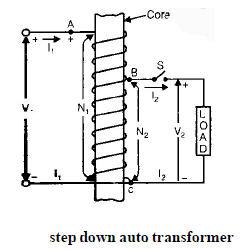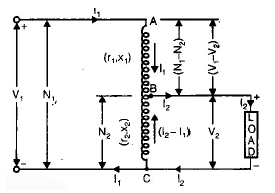Number of turns of primary winding AC = Nand number of turns of secondary windings BC = N2

• If applied voltage Vacross AC winding, then the Voltage across winding BC is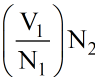• If ideal case, Input power = output power

V1I1 cos θ1 = V2I2 cos θ2

• If cos θ1 = cos θ2

then, V1I1 = V2I2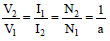Where a = turn ratio.

Advantages of Auto trasformer Over Two Winding Transformer
• Auto transformer ha higher efficiency than two winding of same output.
• An autotransformer has lower value of leakage impedance and has superior voltage regulation than two winding transformer of same output.
• An auto transformer is more economic.

Disadvantages of Autotransformer Over Two Winding Transformer
• If turn ratio (a) differs for from unity the economic advantage of autotransformer over two winding transformer decreases.
• The short circuit current in an auto transformer is higher than that in a corresponding two winding transformer.
• The main disadvantage of an auto transformer is due to the direct electric connection between low voltage and high voltage side. If primary is supplied at high voltage, then an open circuit in common winding, would result in the appearance of dangerously high voltage on low voltage side.

Comparison of Characteristic of Autotransformers and Two Winding Transformers

Copper Saving in Autotransformer

• Cu(auto) = Cu(2wdg)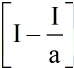• Percentage copper saving
% Cu saving = 1/a x 100

Ratings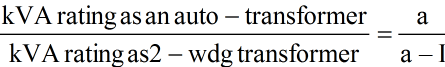Losses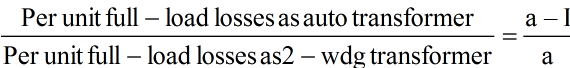Voltage Regulation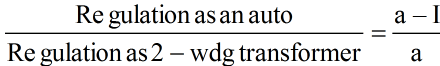Short Circuit Current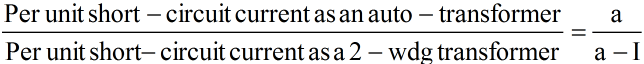Note:
• Single phase and 3 phase auto transformer are mainly employed.
• For interconnecting power systems having voltage ration, not differing from unity.
• For obtaining variable output voltages.

PARALLEL OPERATION OF 1-Ø TRANSFORMER
Advantage of Parallel operation
There are following advantages of the parallel operation of single-phase transformer

• With two or more transformers the power system become more reliable. If one transformer develops fault, it can be removed and the other transformers can maintain the flow of power, though at a reduced level.
• Transformers can be switched-off or on depending upon the power demand. In this manner the transformer losses decreases and the system becomes more economical and efficient in operation.
• The cost of a standby (or spare) unit is much less when two or more transformers are installed.

Condition for Parallel Operation
• Condition for the satisfactory parallel operation of two or more single-phase transformers are as follows.

Necessary Conditions
• The polarities of the transformers must be the same.
• The turn ratios of the transformers should be equal. i.e. with the primaries connected to the same voltage source, the secondary voltages of all transformers should be equal in magnitude.

Desirable Conditions
• The per unit leakage impedance of the transformers based on their own KVA ratings must be equal.
• The ratio of equivalent leakage reactance to equivalent resistance i.e. xeq/req should be equal for all the transformers.

• This condition ensures that both transformers operate at the same power factor, thus sharing active power and reactive volt-amperes according to their ratings.

parallel Operation at Same voltage Ratio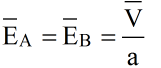Where EA and EB are no-load secondary voltages.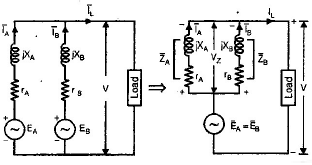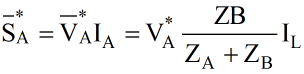or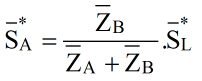Similarly,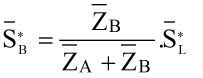For Proportional Load Sharing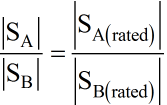or ZA(pu) = ZB(pu)
Where the pu values are on their respective or own base.

Same Voltage Ratio and Different (x/r) Ratio

Let θA > θB
Equal S for two transformer with
SA = S with θA
SB = S with θB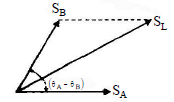Then S=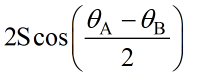Unequal Voltage Ratio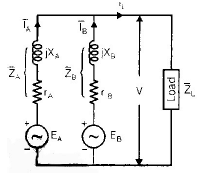• Following steps are followed to calculate load kVA.

Steps:

•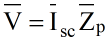•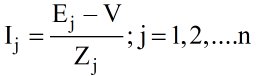•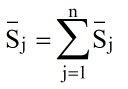where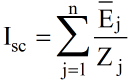and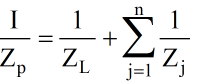THREE PHASE TRANSFORMER
• When three identical units of single-phase transformers are used, the arrangement is commonly called a bank of three transformers or a three- phase transformer bank.
• Three phase current in three primaries produce three-phase fluxes mutually time-displaced by 120°. These fluxes flow through their respective
yokes and then through the central limbs placed together. The resultant flux in the three central limbs must be zero.

Core Type Transformer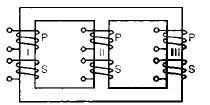Three-phase core-type transformer from three single-phase units
• The reluctance offered to the flux of central limb II is less than the reluctance offered to the outer two fluxes produced in legs I and III. Consequently, the exciting current for the phase winding on the central limb is less than it is for the outer two phase windings. Shell Type Transformer
• A three- phase shell type transformer is obtained if three single-phase shell type cores are placed side by side.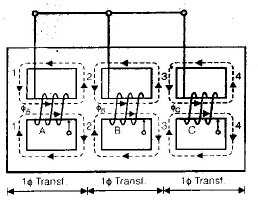Three-phase shell type transformer with three windings wound in the same direction
• In the magnetic circuits marked 2 and 3, the resultant flux is seen to be equal to the phasor difference of the component fluxes.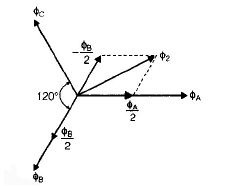• For the same flux density, the cross-sectional areas at 2 and 3 are 86% of the central core area.

parallel Operation of 3-ø Transformers
• The various conditions that must be fulfilled, for the successful parallel operation of 3-phae transformers, are as follows:
• The line voltage rations of the transformers must be the same.
• The transformers should have equal per unit leakage impedances.
• The ratio of equivalent leakage reactance to equivalent resistance should be same for all the transformers.

• The transformers should have the same polarity.
• In addition to these four conditions, two more essential conditions that must be fulfilled for the parallel operation of three-phase transformers are as follows:

Relative phase displacement: The relative phase displacement between the secondary line voltage of all the transformers must be zero i.e. the transformers to be connected in parallel, must belong to the same group number.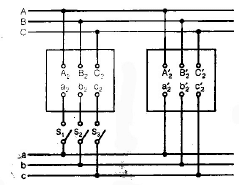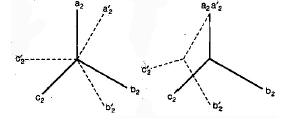Note:
• Transformers for group numbers 3 and 4 can be successfully operated in parallel.
• Phase Sequence: An improper phase sequence as shown in figure (b) below, would give zero voltage across switch S1 and line voltages across switch S2 and S3. Consequently the parallel operations is not possible.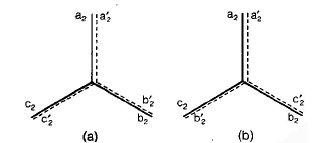The document Transformers - 3 Notes | Study Electrical Engineering SSC JE (Technical) - Electrical Engineering (EE) is a part of the Electrical Engineering (EE) Course Electrical Engineering SSC JE (Technical).
All you need of Electrical Engineering (EE) at this link: Electrical Engineering (EE)

## Electrical Engineering SSC JE (Technical)

2 videos|65 docs|37 tests
 Use Code STAYHOME200 and get INR 200 additional OFF

## Electrical Engineering SSC JE (Technical)

2 videos|65 docs|37 tests

Track your progress, build streaks, highlight & save important lessons and more!

,

,

,

,

,

,

,

,

,

,

,

,

,

,

,

,

,

,

,

,

,

;